# Sheet1 Control 1. Solve the following differential equations using Laplace transforms. Assume zero initial conditions dx...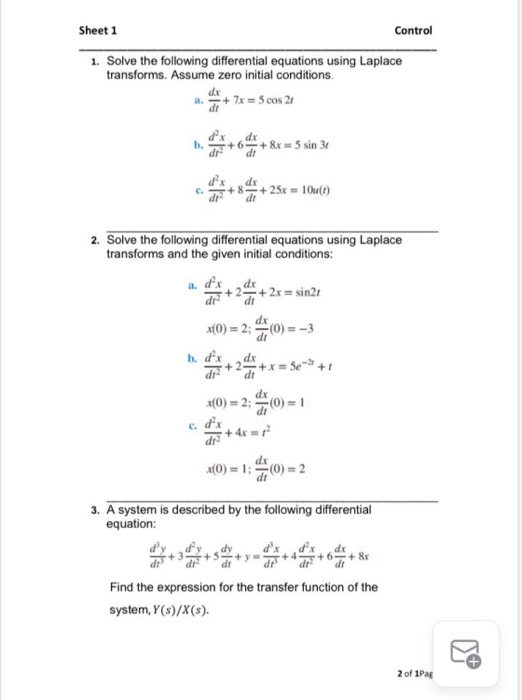Sheet1 Control 1. Solve the following differential equations using Laplace transforms. Assume zero initial conditions dx + 7x = 5 cos 21 di b. + 6 + 8x = 5 sin 31 dt + 25x = 10u(1) 2. Solve the following differential equations using Laplace transforms and the given initial conditions: de *(0) = 2 () = -3 dx +2+2x = sin21 di dx di dx di 7+2 x(0) = 2:0) = 1 ed + 4x x(0) = 1:0) = 2 3. A system is described by the following differential equation: x +4 +6 dr + & Find the expression for the transfer function of the system, Y(s)/X(s). 2 of 1Pag

Here is the solution....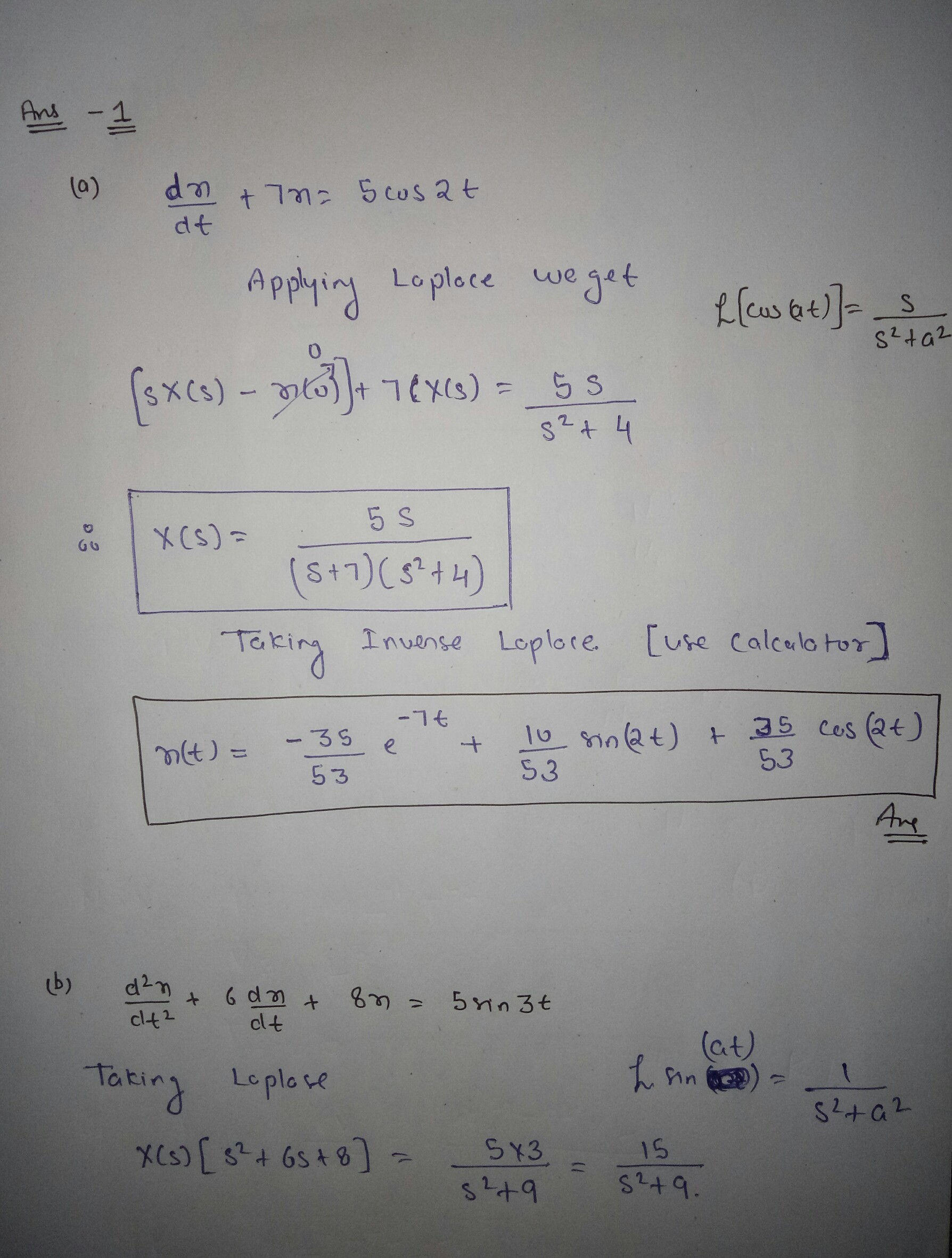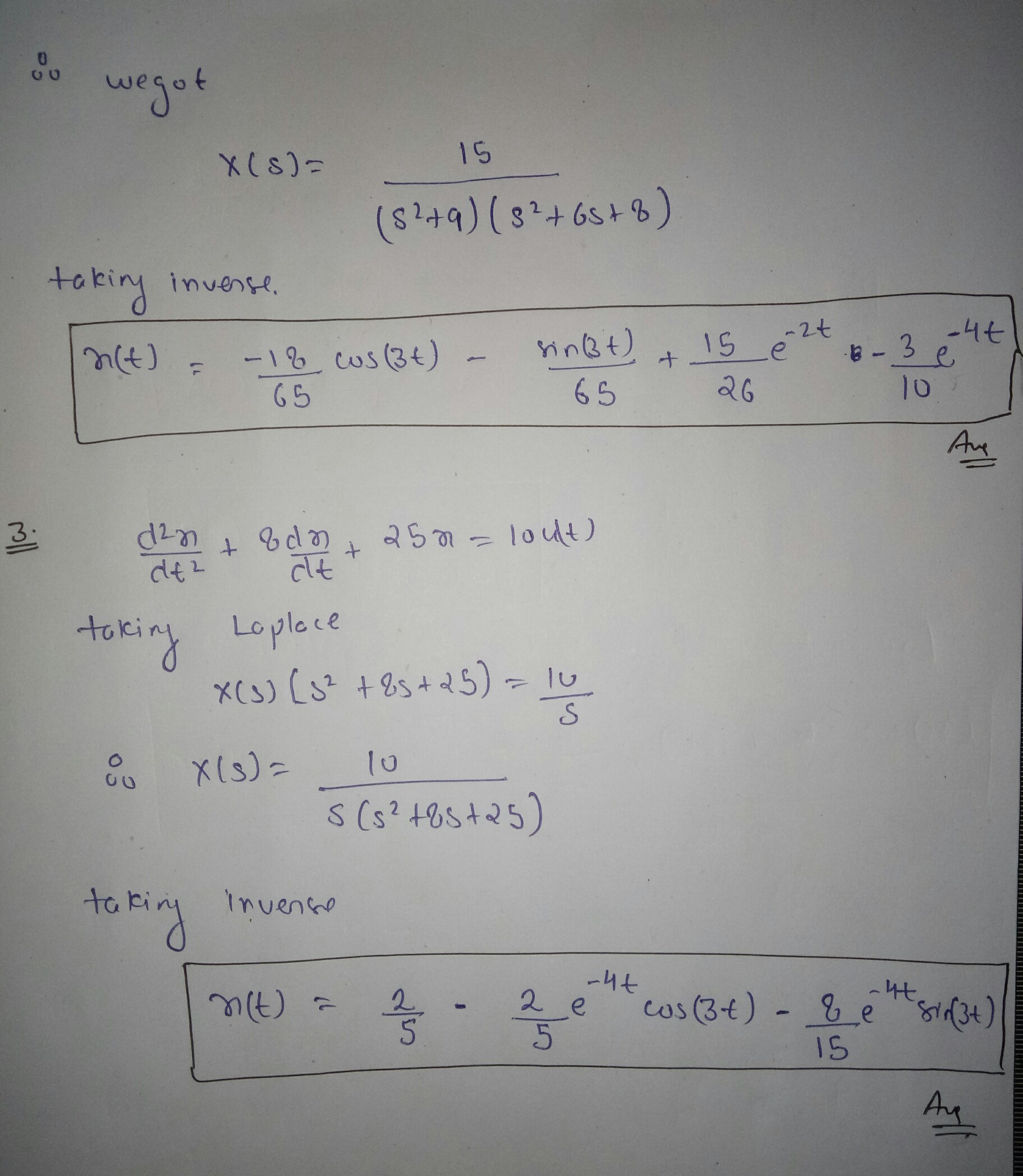As per the guidelines I have allowed to do only one question as you have not mentioned so I did the first one..... I hope you understand it...

#### Earn Coin

Coins can be redeemed for fabulous gifts.

Similar Homework Help Questions
• ### use Laplace transforms to solve the given system of differential equations ponts) 6)) Use Laplace transforms...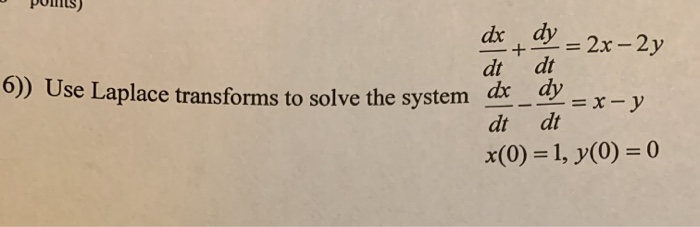use Laplace transforms to solve the given system of differential equations ponts) 6)) Use Laplace transforms to solve the system dc y = 2x-2y dt.dt dx _ ay = x - y dt at x(O) = 1, y(0) = 0

• ### Need help with this: Solve the following system of differential equations by using Laplace transforms. with...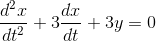Need help with this: Solve the following system of differential equations by using Laplace transforms. with x(0) = 0, x'(0) = 2, and y(0) = 0. Thank you for your time and help! dr 22 +3 + 3y = 0 d2dt dx2 + 3y = tet

• ### Solve the system of equations with Laplace Transforms: (differential equations) all parts please Solve the system...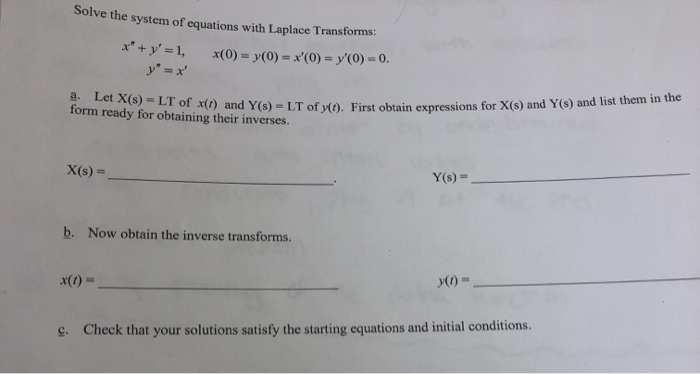Solve the system of equations with Laplace Transforms: (differential equations) all parts please Solve the system of equations with Laplace Transforms: x' + y' = 1, x(0) = y(0) = x'(0) = y'(0) = 0. y" = x' Let X(s) = LT of x(t) and Y(s) = LT of y(1). First obtain expressions for X(s) and Y(s) and list them in the form ready for obtaining their inverses. a. Y(s) = X(s) = %3D b. Now obtain the inverse transforms....

• ### write MATLAB scripts to solve differential equations. Computing 1: ELE1053 Project 3E:Solving Differential Equations Project Principle...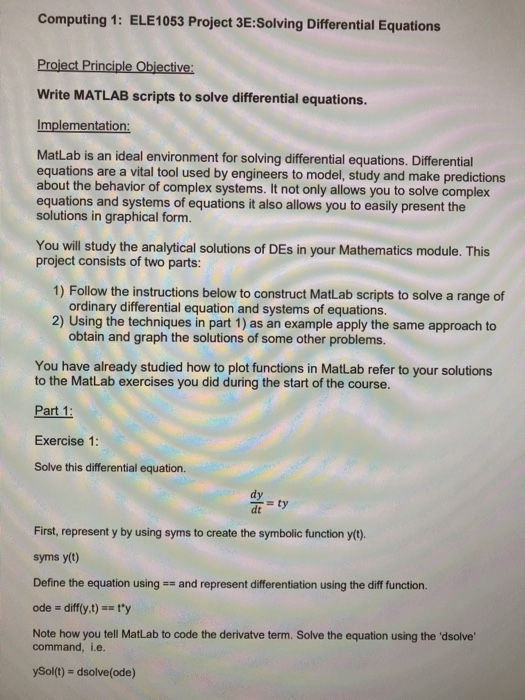write MATLAB scripts to solve differential equations. Computing 1: ELE1053 Project 3E:Solving Differential Equations Project Principle Objective: Write MATLAB scripts to solve differential equations. Implementation: MatLab is an ideal environment for solving differential equations. Differential equations are a vital tool used by engineers to model, study and make predictions about the behavior of complex systems. It not only allows you to solve complex equations and systems of equations it also allows you to easily present the solutions in graphical form....

• ### Solve the system of differential equations using Laplace transformation dx dy dt - x = 0,...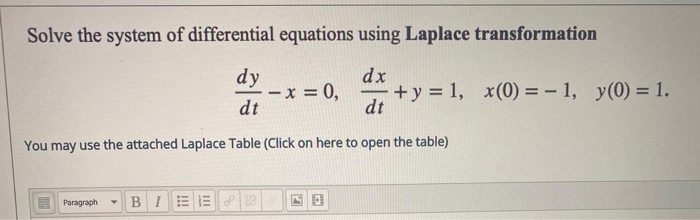Solve the system of differential equations using Laplace transformation dx dy dt - x = 0, + y = 1, x(0) = -1, y(0) = 1. dt You may use the attached Laplace Table (Click on here to open the table) Paragraph В І

• ### Solve the following differential equation using the Laplace transform and assuming the given initial conditions. [Note:...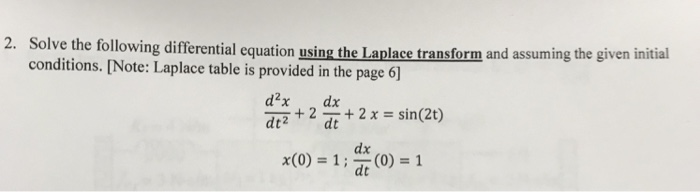Solve the following differential equation using the Laplace transform and assuming the given initial conditions. [Note: Laplace table is provided in the page 6] dt2 dt dix x(0) = 1 ; (0) = 1 dt

• ### use the Laplace transform to solve the given system of differential equations dx dt dx dt...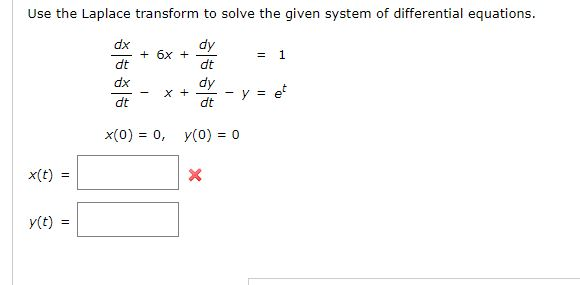use the Laplace transform to solve the given system of differential equations dx dt dx dt dt dt x(0) 0, y(o)0 x(t) =

• ### Solve the system of differential equations dx/dt = x-y, dy/dt = 2x+y subject to the initial conditions x(0)= 0 and y(0) = 1.

Solve the system of differential equations dx/dt = x-y, dy/dt = 2x+y subject to the initial conditions x(0)= 0 and y(0) = 1.

• ### K1=10, k2=7. Please do both 3) Using Laplace transforms, solve the following differential equations with the...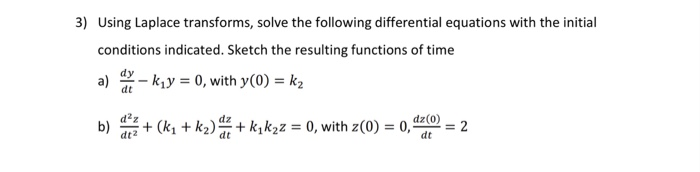K1=10, k2=7. Please do both 3) Using Laplace transforms, solve the following differential equations with the initial conditions indicated. Sketch the resulting functions of time dy 0, with y(O) - k2 bk2)+ kik20, with z(0) - 0, t( 2 dt2

• ### 2. Using Laplace transform, solve the system of differential equations d.x: dy dt where x(0)1 2. Using Laplace transform, solve the system of differential equations d.x: dy dt where x(0)1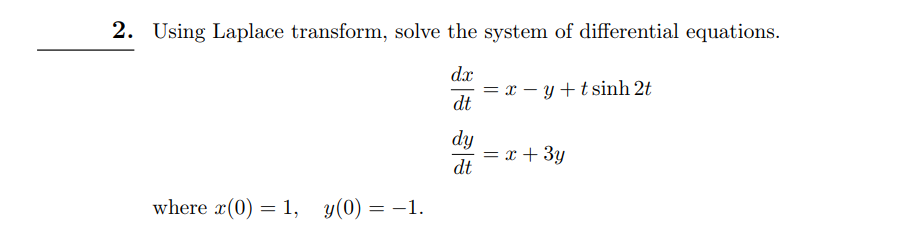2. Using Laplace transform, solve the system of differential equations d.x: dy dt where x(0)1 2. Using Laplace transform, solve the system of differential equations d.x: dy dt where x(0)1